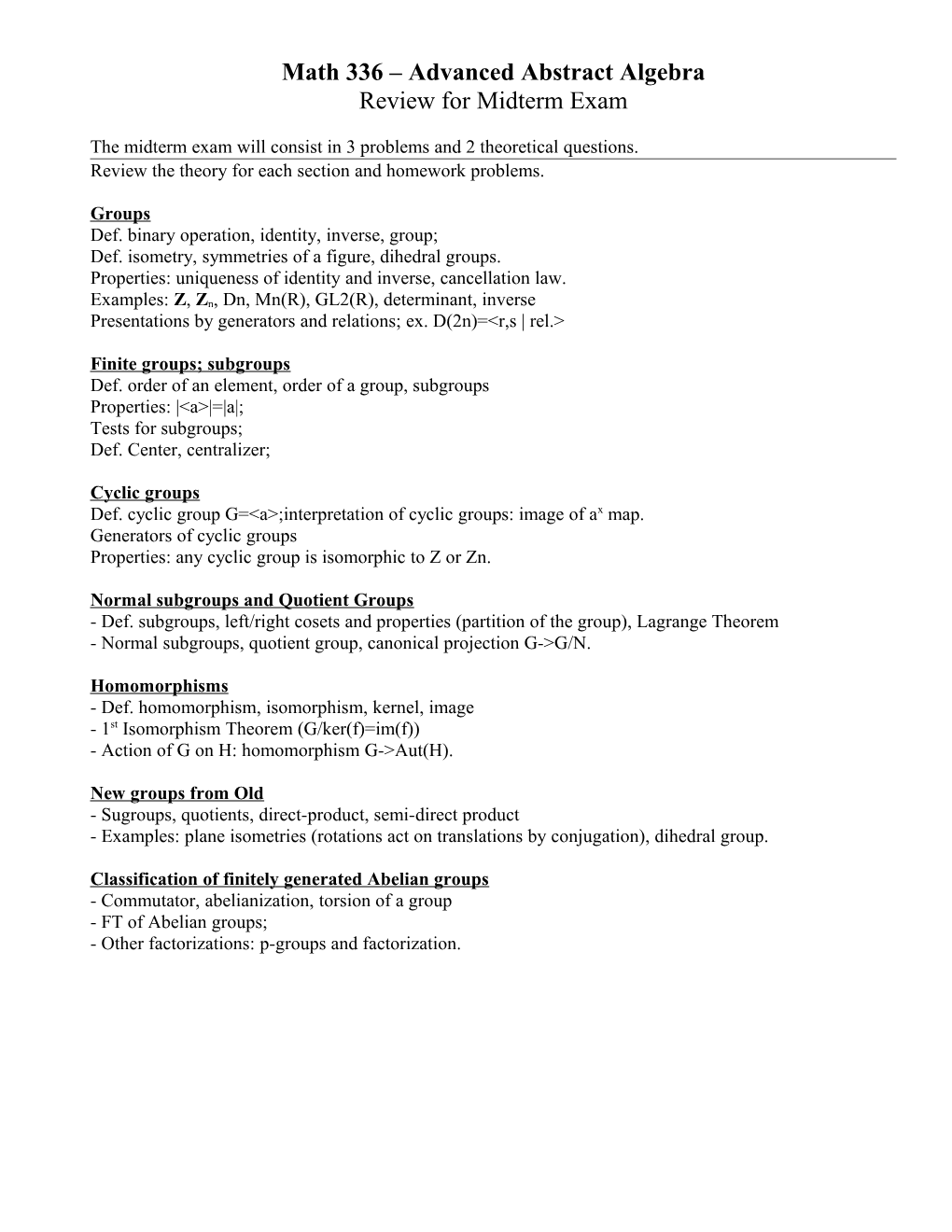# Math 336 Advanced Abstract AlgebraMath 336 – Advanced Abstract Algebra

Review for Midterm Exam

The midterm exam will consist in 3 problems and 2 theoretical questions.

Review the theory for each section and homework problems.

Groups

Def. binary operation, identity, inverse, group;

Def. isometry, symmetries of a figure, dihedral groups.

Properties: uniqueness of identity and inverse, cancellation law.

Examples: Z, Zn, Dn, Mn(R), GL2(R), determinant, inverse

Presentations by generators and relations; ex. D(2n)=<r,s | rel.>

Finite groups; subgroups

Def. order of an element, order of a group, subgroups

Properties: |<a>|=|a|;

Tests for subgroups;

Def.Center, centralizer;

Cyclic groups

Def. cyclic group G=<a>;interpretation of cyclic groups: image of ax map.

Generators of cyclic groups

Properties: any cyclic group is isomorphic to Z or Zn.

Normal subgroups and Quotient Groups

- Def. subgroups, left/right cosets and properties (partition of the group), Lagrange Theorem

- Normal subgroups, quotient group, canonical projection G->G/N.

Homomorphisms

- Def. homomorphism, isomorphism, kernel, image

- 1st Isomorphism Theorem (G/ker(f)=im(f))

- Action of G on H: homomorphism G->Aut(H).

New groups from Old

- Sugroups, quotients, direct-product, semi-direct product

- Examples: plane isometries (rotations act on translations by conjugation), dihedral group.

Classification of finitely generated Abelian groups

- Commutator, abelianization, torsion of a group

- FT of Abelian groups;

- Other factorizations: p-groups and factorization.

Math 336 – Review for Final Exam

Final exam will consist in 3 problems and 2 theoretical questions.

Review the theory for each section and homework problems.

Abelian groups and codes

- Hamming code, distance, block code, parity check code

- Linear encoding, dual code

Sylow’s Theorems

- 1,2& 3rd Syllow Theorem

- Cauchy theorem

Permutations (Set automorphisms)

- Permutations of a set Aut(S), cycles, transpositions, composition;

- Th. Aut(S) is a group (with proof); symmetric group Sn;

- Order of a cycle, disjoint cycles commute;

- Factorization into cycles.

- Factorization into transpositions; length of product unique mod 2; even / odd permutations;

- Alternating group An

- Conjugacy classes: definition, characterization: correspond to shapes of permutations (partitions of n).

Galois Theory

- Fields, field extensions, degree [E:F] is multiplicative, adjoining a root

- Automorphisms of a field, automorphism of a field extension,

- Separable polynomials, irreducible polynomials

- Splitting fields and properties [E:F]<= deg(P)!

- Galois extensions, Galois groups Aut(E/F) for a normal extension

- Degree of a splitting field [E:F]=|Aut(E/F)|

- Galois correspondence: fields <-> subgroups

- Applications to higher order polynomial equations: Cardano’s method; deg >=5 not solvable;